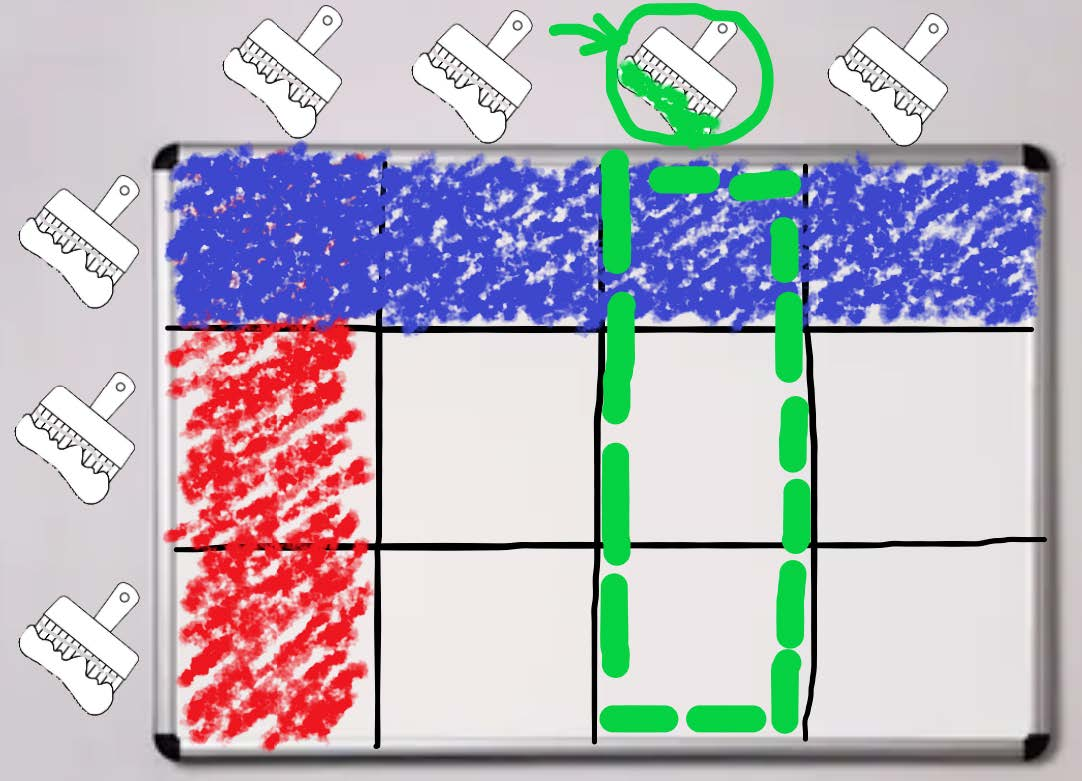# #795. 【NOI 春季测试 2023】涂色游戏• 如果 $opt_i=0$，那么这次操作会将第 $x_i$ 涂成颜色 $c_i$。
• 如果 $opt_i=1$，那么这次操作会将第 $x_i$ 涂成颜色 $c_i$。

### 样例一

#### input

2
5 5 9
1 5 1
0 4 0
1 4 1
0 3 0
1 3 1
0 2 0
1 2 1
0 1 0
1 1 1
3 3 3
0 1 2
0 3 1
1 1 3

#### output

1 0 0 0 0
1 1 0 0 0
1 1 1 0 0
1 1 1 1 0
1 1 1 1 1
3 2 2
3 0 0
3 1 1

### 子任务

• $1 \leq T \leq 10$，$1 \leq n,m \leq 10^5$，$0 \leq q \leq 10^5$，$0 \leq c_i \leq 10^9$。
• 若 $opt_i=0$，则 $1 \leq x_i \leq n$；若 $opt_i=1$，则 $1 \leq x_i \leq m$。
• 单个测试点中所有数据的 $n \cdot m$ 的总和不超过 $10^6$，$q$ 的总和不超过 $10^6$。

1 $1$ $1$ $0$
2 $1$
3 $10$ $20$
4 $10^5$ $10^5$
5
6
7 $10$ $10$ $20$
8 $50$ $50$ $100$
9
10 $1000$ $1000$ $2000$
11
12
13 $10^5$
14
15 $10^5$ $10^5$
16
17
18
19
20